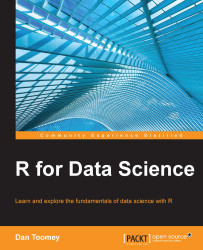•#### R for Data Science#### Overview of this book

R for Data ScienceCreditswww.PacktPub.comPrefaceFree Chapter
Data Mining PatternsData Mining SequencesText MiningData Analysis – Regression AnalysisData Analysis – CorrelationData Analysis – ClusteringData Visualization – R GraphicsData Visualization – PlottingData Visualization – 3DMachine Learning in ActionPredicting Events with Machine LearningSupervised and Unsupervised LearningIndex## Association rules

Association rules describe associations between two datasets. This is most commonly used in market basket analysis. Given a set of transactions with multiple, different items per transaction (shopping bag), how can the item sales be associated? The most common associations are as follows:

• Support: This is the percentage of transactions that contain A and B.

• Confidence: This is the percentage (of time that rule is correct) of cases containing A that also contain B.

• Lift: This is the ratio of confidence to the percentage of cases containing B. Please note that if lift is 1, then A and B are independent.

### Mine for associations

The most widely used tool in R from association rules is `apriori`.

#### Usage

The `apriori` rules library can be called as follows:

`apriori(data, parameter = NULL, appearance = NULL, control = NULL)`

The various parameters of the `apriori` library are explained in the following table:

Parameter

Description

`data`

This is the transaction data.

`parameter`

This stores the default behavior to mine, with `support` as 0.1, `confidence` as 0.8, and `maxlen` as 10. You can change parameter values accordingly.

`appearance`

This is used to restrict items that appear in rules.

`control`

This is used to adjust the performance of the algorithm used.

#### Example

You will need to load the `apriori` rules library as follows:

```> install.packages("arules")
> library(arules)```

`> data <- read.csv("http://www.salemmarafi.com/wp-content/uploads/2014/03/groceries.csv")`

Then, we can generate rules from the data as follows:

```> rules <- apriori(data)

parameter specification:
confidenceminvalsmaxaremavaloriginalSupport support minlenmaxlen target
0.8    0.1    1 none FALSE            TRUE     0.1      1     10  rules
ext
FALSE

algorithmic control:
filter tree heap memopt load sort verbose
0.1 TRUE TRUE  FALSE TRUE    2    TRUE

apriori - find association rules with the apriori algorithm
version 4.21 (2004.05.09)        (c) 1996-2004   Christian Borgelt
set item appearances ...[0 item(s)] done [0.00s].
set transactions ...[655 item(s), 15295 transaction(s)] done [0.00s].
sorting and recoding items ... [3 item(s)] done [0.00s].
creating transaction tree ... done [0.00s].
checking subsets of size 1 2 3 done [0.00s].
writing ... [5 rule(s)] done [0.00s].
creating S4 object  ... done [0.00s].```

There are several points to highlight in the results:

• As you can see from the display, we are using the default settings (confidence 0.8, and so on)

• We found 15,000 transactions for three items (picked from the 655 total items available)

• We generated five rules

We can examine the rules that were generated as follows:

```> rules

set of 5 rules
> inspect(rules)

lhsrhs              support confidence     lift
1 {semi.finished.bread=} => {margarine=}   0.2278522          1 2.501226
3 {margarine=}           => {ready.soups=} 0.3998039          1 1.861385
`> rules <- apriori(data, parameter = list(supp = 0.001, conf = 0.8))`